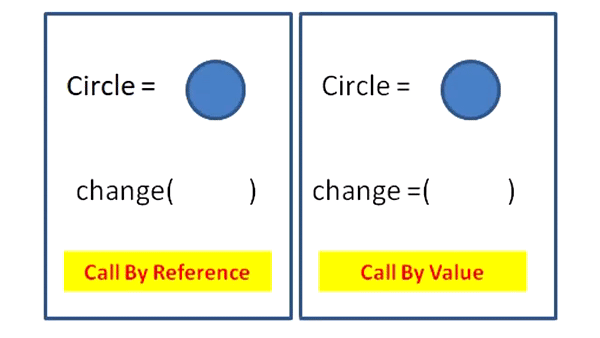×

Search anything:

# Pass Variable By Reference in Python

#### Software Engineering PythonIndian Technical Authorship Contest starts on 1st July 2023. Stay tuned.

In this article, we have explored how to pass a variable by reference to a function in Python. We have demonstrated the ideas by Python code snippets.

There a two types of passing parameters to a function in Python:

1. Pass By Value
2. Pass By Reference

## Pass By Value:

A copy of the argument passed to the function is made and when any operation is done on the copy the actual value does not change. It only changes the value of the copy which is made inside the function.

``````def fun(a):
a+=10
print("Inside function call",a)

a=20
print("Before function call",a)
fun(a)
print("After function call",a)
``````

Output:

``````Before function call 20
Inside function call 30
After function call 20
``````

Explanation:

Here a copy of the argument is made and changes are made to that copy so it does not affect the original value.So after the function call it prints the original value.

## Pass By Reference:

In this the reference to the original the arguments is passed and when any operation is performed on the argument the actual value also changes. It changes the value in both function and globally.

``````def fun(a):
a.append('i')
print("Inside function call",a)

a=['H']
print("Before function call",a)
fun(a)
print("After function call",a)
``````

Output:

``````Before function call ['H']
Inside function call ['H', 'i']
After function call ['H', 'i']
``````

Explanation:
Here as a list is used it's reference is passed into the function when any changes are made to the list its effect is shown even after the function call.

Below example gives a detailed explanation of both:Python actually uses Call By Object Reference also called as Call By Assignment which means that it depends on the type of argument being passed.

1. If immutable objects are passed then it uses Call by value
2. If mutable objects are passed then it uses Call by reference

## Immutable Objects:

These objects cannot change their state or value.Different types of immutable objects are:

• String
• Integer
• Tuple
``````def fun(s,num,d):
s="Hello"
num=10
d=(5,6,7)
print("Inside function call",s,num,d)
s="Opengenus"
num=1
d=(1,2,3)
print("Before function call",s,num,d)
fun(s,num,d)
print("After function call",s,num,d)
``````

Output:

``````Before function call Opengenus 1 (1, 2, 3)
Inside function call Hello 10 (5, 6, 7)
After function call Opengenus 1 (1, 2, 3)
``````

Explanation:
Even though the values of s,num,d are changed inside the function the original value does not get changed as they are passed by value. Due to this it prints the same value even after function call.

## Mutable Objects:

These objects can change their state or value.Different types of mutable objects are:

• List
• Dictionary
• Set
``````def fun(s,num,d):
s.append(5)
num["name"]="harry"
d|=set({1,2})
print("Inside function call",s,num,d)

s=[1,2,4]
num={"name":"john"}
d={4,5,6}

print("Before function call",s,num,d)

fun(s,num,d)

print("After function call",s,num,d)
``````

Explanation:
Here the mutable objects like dictionary,list,set are passed into the function and their values are modified in it. After the function call the original value is updated with the new value.

## Passing an Immutable Object By Reference:

As mentioned earlier whenever immutable object is passed to a function it is passed as call by value. But this behaviour can be changed by some different ways as follows:

### Returning and Reassigning:

In this approach the value is changed in the called function and then is returned. This returned value is assigned to the original variable.

``````def ref(s):
s+="Opengenus"
return s

s="Hello "
print("Before passing to function -",s)
s=ref(s)
print("After passing to function -",s)
``````

Output:
Before passing to function - Hello
After passing to function - Hello Opengenus

Explanation:
Here the string is modified in the function ref() and is returned.This returned value is reassigned to the variable "s" so that it contains the modified value.

### Using Class and self keyword:

In this way the self keyword is used which is used to access instance variables within a class.

``````class First():
def __init__(self):
self.val=1
print("Before calling function",self.val)
self.modify(self.val)
print("After calling function",self.val)

def modify(self,n):
self.val=n+1

f=First()
``````

Output:
Before calling function 1
After calling function 2

Explanation:
Here instance variable val is changed inside the modify() function. As the instance variable is reassigned the value so after calling the function the original value gets changed.

### Passing Immutable objects inside Dictionaries or Lists:

Immutable objects are passed inside mutable objects like dictionaries or lists or sets as parameters to function so that they can be modified within the function.

``````def change_dict(d):
d["hello"]="First Article"

def change_list(l):
l=l+5

d={"hello":"opengenus"}
l=

print("Before passing to function values are ")
print("Dictionary - ",d)
print("List -",l)

change_dict(d)
change_list(l)

print("After passing to function values are ")
print("Dictionary - ",d)
print("List -",l)
``````

Output:
Before passing to function values are
Dictionary - {'hello': 'opengenus'}
List - 
After passing to function values are
Dictionary - {'hello': 'First Article'}
List - 

Consider the following Python code for an interesting question:

``````class Article:
def __init__(self,val=2):
self.val=val
self.change(10)
def change(self,n):
self.val+=n
f=Article(10)
print("With argument",f.val)
e=Article()
print("Without argument",e.val)
``````

## Question

#### Output of the above code snippet

With argument 10; Without argument 12
With argument 20; Without argument 12
With argument 12; Without argument 20
None of the above
Initially 10 is sent as argument to class and 10 is added and when argument is not sent 2 is initialized and 10 is added again.